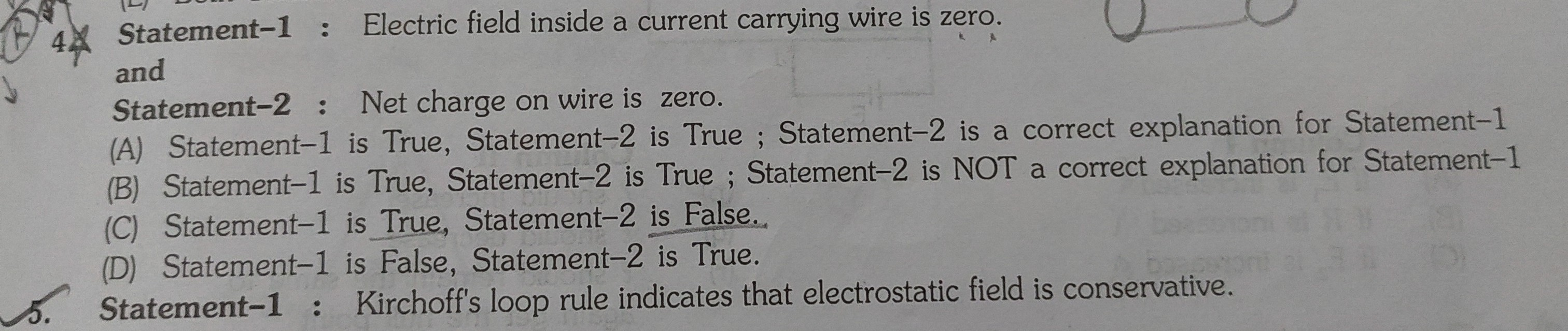# Doubt from current electricityIs the answer Option (D) ?

1 Like

I think that the answer should be option C

Net electric field inside any conductor is always zero.

I think that it should be C because

What happens is when a wire is connected in a circuit , a potential difference across it is generated and thus there is an electric field and due to this electric field electron starts to in opposite direction of electric field and thus of suppose electric field was towards right then electron gets accumulated towards left which leads to a electron deficient region towards right and thus induced electric field gets generated towards left .. this process continues till Eext=Einduced and thus final electric field inside = 0
There are many evidences of this fact

1. if E field would have been there , there would have been a potential drop when we move across the conducting wire , but we know V = const throughout a wire
2. If E would have been there then electrons would have accelerated and thus value of current would not have been constant
3. Electric field inside any conductor = 0

Also I don’t think net charge should be considered zero in a wire as there are only electrons

@Shwetanshu_2018 how you are saying that there are only electros inside conducting wire.

It should be net charge on a current carrying wire, imo. If wire is connected to a circuit then net charge is non-zero and if it it isn't connected net charge will be zero.

@Lavesh_2020 I think it is asking about current carrying wire only , Usually such questions are assertions reasoning type , so i think it must be about current carrying wire instead of just an isolated wire

@Ankith_2020 i think i did a mistake there , i meant to write something else .
But i want to change my answer a bit , i think option B should be correct As
Electric current is the flow of free electrons in the conductor. At any instant, the number of electrons leaving the wire is always equal to the number of electrons flowing from the battery into it. Hence, the net charge on the wire is zero.

If we’ll say that the current carrying conductor is charged, it will violate the Kirchoff junction rule, which is based on the fact that in an electric circuit, a point can neither act as a source of charge, nor can the charge accumulate at that point.

1 Like

shwetanshu 2018 why you didn't go with correct explaination

i am satisfied with the logic for statement 2 to be correct

I did not quite get what you mean by that!

I can’t find a reason for electric field to be non zero inside a wire !Electric field inside a current carrying conductor is zero as the charges inside it distributes themselves on the surface of the conductor. The net charge in the current carrying wire is zero. Thus the assertion is true while the reason is wrong.

do you mean that
reason is wrong because there is no mention of current carrying wire,and obviously a wire can have some charge

Reason is given for an assertion statement it is wrong because net charge one the surface of the wire which can be zero and can be non zero. And electric field inside the wire is not related to charge on the outside surface so the reason is wrong.

The given answer is correct as current carrying wire can never be acts as a pure conductor and there is some resistance always present which support flowing of current. Hence net electric field inside conductor can never be zero.